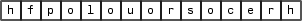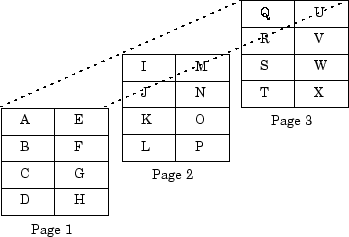## MATLAB Data

### The MATLAB Array

The MATLAB® language works with a single object type: the MATLAB array. All MATLAB variables (including scalars, vectors, matrices, character arrays, cell arrays, structures, and objects) are stored as MATLAB arrays. In C/C++, the MATLAB array is declared to be of type `mxArray`. The `mxArray` structure contains the following information about the array:

• Its type

• Its dimensions

• The data associated with this array

• If numeric, whether the variable is real or complex

• If sparse, its indices and nonzero maximum elements

• If a structure or an object, the number of fields and field names

To access the `mxArray` structure, use functions in the C or Fortran Matrix APIs. These functions allow you to create, read, and query information about the MATLAB data in your MEX files. Matrix APIs use the `mwSize` and `mwIndex` types to avoid portability issues and allow MEX source files to be compiled correctly on all systems.

### Lifecycle of mxArray

Like MATLAB functions, a MEX-file gateway routine passes MATLAB variables by reference. However, these arguments are C pointers. A pointer to a variable is the address (location in memory) of the variable. MATLAB functions handle data storage for you automatically. When passing data to a MEX-file, you use pointers, which follow specific rules for accessing and manipulating variables. For information about working with pointers, refer to a programming reference, such as The C Programming Language by Kernighan, B. W., and D. M. Ritchie.

Note

Since variables use memory, you need to understand how your MEX-file creates an `mxArray` and your responsibility for releasing (freeing) the memory. This is important to prevent memory leaks. The lifecycle of an `mxArray`—and the rules for managing memory—depends on whether it is an input argument, output argument, or local variable. The function you call to deallocate an `mxArray` depends on the function you used to create it. For more information, look up the functions to create arrays in the C Matrix API.

#### Input Argument `prhs`

An `mxArray` passed to a MEX-file through the `prhs` input parameter exists outside the scope of the MEX-file. Do not free memory for any `mxArray` in the `prhs` parameter. Also, `prhs` variables are read-only; do not modify them in your MEX-file.

#### Output Argument `plhs`

If you create an `mxArray` (allocate memory and create data) for an output argument, the memory and data exist beyond the scope of the MEX-file. Do not free memory on an `mxArray` returned in the `plhs` output parameter.

#### Local Variable

You allocate memory whenever you use an `mxCreate*` function to create an `mxArray` or when you call the `mxCalloc` and associated functions. After observing the rules for handling input and output arguments, the MEX-file should destroy temporary arrays and free dynamically allocated memory. To deallocate memory, use either `mxDestroyArray` or `mxFree`. For information about which function to use, see MX Matrix Library.

### Data Storage

MATLAB stores data in a column-major (column-wise) numbering scheme, which is how Fortran stores matrices. MATLAB uses this convention because it was originally written in Fortran. MATLAB internally stores data elements from the first column first, then data elements from the second column second, and so on, through the last column.

For example, given the matrix:

```a = ['house'; 'floor'; 'porch'] ```
```a = house floor porch```

its dimensions are:

```size(a) ```
```ans = 3 5```

and its data is stored as:If a matrix is N-dimensional, MATLAB represents the data in N-major order. For example, consider a three-dimensional array having dimensions `4`-by-`2`-by-`3`. Although you can visualize the data as:MATLAB internally represents the data for this three-dimensional array in this order:

 A B C D E F G H I J K L M N O P Q R S T U V W X 0 1 2 3 4 5 6 7 8 9 10 11 12 13 14 15 16 17 18 19 20 21 22 23

The `mxCalcSingleSubscript` function creates the offset from the first element of an array to the desired element, using N-dimensional subscripting.

### MATLAB Data Types

#### Complex Double-Precision Matrices

The most common data type in MATLAB is the complex double-precision, nonsparse matrix. These matrices are of type `double` and have dimensions `m`-by-`n`, where `m` is the number of rows and `n` is the number of columns. The data is stored as a vector of interleaved, double-precision numbers where the real and imaginary parts are stored next to each other. The pointer to this data is referred to as `pa` (pointer to array). To test for a noncomplex matrix, call `mxIsComplex`.

Before MATLAB Version 9.4 (R2018a), MATLAB used a separate storage representation. The data is stored as two vectors of double-precision numbers—one contains the real data and one contains the imaginary data. The pointers to this data are referred to as `pr` (pointer to real data) and `pi` (pointer to imaginary data), respectively. A noncomplex matrix is one whose `pi` is `NULL`. However, to test for a noncomplex matrix, call `mxIsComplex`.

#### Other Numeric Matrices

MATLAB supports single-precision floating-point and 8-, 16-, 32-, and 64-bit integers, both signed and unsigned.

#### Logical Matrices

The logical data type represents a logical `true` or `false` state using the numbers `1` and `0`, respectively. Certain MATLAB functions and operators return logical `1` or logical `0` to indicate whether a certain condition was found to be true or not. For example, the statement ```(5 * 10) > 40``` returns a logical `1` value.

#### MATLAB`char` Arrays

MATLAB `char` arrays store data as unsigned 16-bit integers. To convert a MATLAB `char` array to a C-style string, call `mxArrayToString`. To convert a C-style string to a `char` array, call `mxCreateString`.

#### Cell Arrays

Cell arrays are a collection of MATLAB arrays where each `mxArray` is referred to as a cell. Cell arrays allow MATLAB arrays of different types to be stored together. Cell arrays are stored in a similar manner to numeric matrices, except the data portion contains a single vector of pointers to `mxArrays`. Members of this vector are called cells. Each cell can be of any supported data type, even another cell array.

#### Structures

A `1`-by-`1` structure is stored in the same manner as a `1`-by-`n` cell array where `n` is the number of fields in the structure. Members of the data vector are called fields. Each field is associated with a name stored in the `mxArray`.

#### Objects

Objects are stored and accessed the same way as structures. In MATLAB, objects are named structures with registered methods. Outside MATLAB, an object is a structure that contains storage for an additional class name that identifies the name of the object.

#### Multidimensional Arrays

MATLAB arrays of any type can be multidimensional. A vector of integers is stored where each element is the size of the corresponding dimension. The storage method of the data is the same as for matrices.

#### Empty Arrays

MATLAB arrays of any type can be empty. An empty `mxArray` is one with at least one dimension equal to zero. For example, a double-precision `mxArray` of type `double`, where `m` and `n` equal 0 and `pa` is `NULL`, is an empty array.

### Sparse Matrices

Sparse matrices have a different storage convention from full matrices in MATLAB. The parameter `pa` is still an array of double-precision numbers or logical values, but this array contains only nonzero data elements.

There are three additional parameters: `nzmax`, `ir`, and `jc`. Use the `mwSize` and `mwIndex` types when declaring variables for these parameters.

• `nzmax` is an integer that contains the length of `ir` and `pa`. It is the maximum number of nonzero elements in the sparse matrix.

• `ir` points to an integer array of length `nzmax` containing the row indices of the corresponding elements in `pa`.

• `jc` points to an integer array of length `n+1`, where `n` is the number of columns in the sparse matrix. In C, the first element of an `mxArray` has an index of 0. The `jc` array contains column index information. If the `j`th column of the sparse matrix has any nonzero elements, `jc[j]` is the index into `ir` and `pa` of the first nonzero element in the `j`th column. Index `jc[j+1] - 1` contains the last nonzero element in that column. For the `j`th column of the sparse matrix, `jc[j]` is the total number of nonzero elements in all preceding columns. The last element of the `jc` array, `jc[n]`, is equal to `nnz`, the number of nonzero elements in the entire sparse matrix. If `nnz` is less than `nzmax`, more nonzero entries can be inserted into the array without allocating more storage.

### Using Data Types

You can write source MEX files, MAT-file applications, and engine applications in C/C++ that accept any class or data type supported by MATLAB (see Data Types). In Fortran, only the creation of double-precision `n`-by-`m` arrays and strings are supported. You use binary C/C++ and Fortran MEX files like MATLAB functions.

Caution

MATLAB does not check the validity of MATLAB data structures created in C/C++ or Fortran using one of the Matrix Library create functions (for example, `mxCreateStructArray`). Using invalid syntax to create a MATLAB data structure can result in unexpected behavior in your C/C++ or Fortran program.

#### Declaring Data Structures

To handle MATLAB arrays, use type `mxArray`. The following statement declares an `mxArray` named `myData`:

`mxArray *myData;`

To define the values of `myData`, use one of the `mxCreate*` functions. Some useful array creation routines are `mxCreateNumericArray`, `mxCreateCellArray`, and `mxCreateCharArray`. For example, the following statement allocates an `m`-by-`1` floating-point `mxArray` initialized to `0`:

`myData = mxCreateDoubleMatrix(m, 1, mxREAL);`

C/C++ programmers should note that data in a MATLAB array is in column-major order. (For an illustration, see Data Storage.) Use the MATLAB `mxGet*` array access routines to read data from an `mxArray`.

#### Manipulating Data

The `mxGet*` array access routines get references to the data in an `mxArray`. Use these routines to modify data in your MEX file. Each function provides access to specific information in the `mxArray`. Some useful functions are `mxGetDoubles`, `mxGetComplexDoubles`, `mxGetM`, and `mxGetString`. Many of these functions have corresponding `mxSet*` routines to allow you to modify values in the array.

The following statements read the input `prhs` into a C-style string `buf`.

```char *buf; int buflen; int status; buflen = mxGetN(prhs)*sizeof(mxChar)+1; buf = mxMalloc(buflen); status = mxGetString(prhs, buf, buflen); ```

#### `explore` Example

There is an example source MEX file included with MATLAB, called `explore.c`, that identifies the data type of an input variable. The source code for this example is in `matlabroot``/extern/examples/mex`, where `matlabroot` represents the top-level folder where MATLAB is installed on your system.

Note

In platform-independent discussions that refer to folder paths, this documentation uses the UNIX® convention. For example, a general reference to the `mex` folder is `matlabroot``/extern/examples/mex`.

To build the example MEX file, first copy the file to a writable folder on your path.

`copyfile(fullfile(matlabroot,'extern','examples','mex','explore.c'),'.','f')`

Use the `mex` command to build the MEX file.

`mex explore.c -R2018a`

Type:

```x = 2; explore(x)```
```------------------------------------------------ Name: prhs Dimensions: 1x1 Class Name: double ------------------------------------------------ (1,1) = 2```

`explore` accepts any data type. Try using `explore` with these examples:

```explore([1 2 3 4 5]) explore 1 2 3 4 5 explore({1 2 3 4 5}) explore(int8([1 2 3 4 5])) explore {1 2 3 4 5} explore(sparse(eye(5))) explore(struct('name', 'Joe Jones', 'ext', 7332)) explore(1, 2, 3, 4, 5) explore(complex(3,4))```